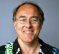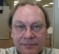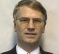## Using noise to increase resolution of ADCStarted by 6 months ago21 replieslatest reply 6 months ago275 views

Hi there -- can you explain in simple terms how – if you are feeding an analog signal into say a 4-bit analog to digital converter (ADC) with 16 digital output states, adding one least-significant bit worth of noise to the analog signal can make it look like you are using a 5-bit ADC?

I used to know this, but now I'm finding it difficult to wrap my brain around.

[ - ]Hello,

You've been given some good references, to which I'll add one more.  It underscores the need for noise addition, in addition to oversampling (4X for each additional bit), as requirement for gaining the addition resolution

I tried to add a picture highlighting some of the key points, from section 3.1 and 3.2, but was unable, so will just quote below:

"For each additional bit of resolution, n, the signal must be oversampled four times"

"However another criteria for a successful enhancement of the resolution is that the input signal has to vary when sampled. This may look like a contradiction, but in this case variation means just a few LSB. The variation should be seen as the noise-component of the signal."

Regards,

Robert

[ - ]Thanks Robert -- much appreciated -- Max

[ - ]Just adding some noise will make it look like a noisy 4 bit converter.

You would also need to average (or otherwise filter) multiple readings together.

There's a not bad Silabs article here:

For lots of complicated reasons it often doesn't work as well as you hope.

Most digital scope can do it and it often works quite well for them

MK

[ - ]Great -- thanks Michael -- Max

[ - ]Hi MaxMax,

it is not just adding noise. Just adding noise to something makes this thing noisier, not better.

You have add noise, oversample and perform averaging on multiple samples in order to get better resolution on a given ADC.

You can find a quite good and simple explanation of the underlying idea of the "adding noise to get better resolution" in chapter 2.4 of the "Principles of Digital Audio" by Ken Pohlmann

Benoit

[ - ]If only I had a copy LOL But thanks for the heads-up :-)

[ - ]@MichaelKellett gave a good summary. It's called dithering, and you need to combine it with oversampling and filtering to increase the effective resolution.

Noiseless ADCs (not truly realistic but you can start there) can be thought of as being the sum of an exact (infinite resolution) and quantization noise that is a function of the input voltage. In most cases you can think of the signal and quantization noise as being uncorrelated; oversampling and filtering helps reduce the effective quantization noise, at the cost of having to get more samples above the Nyquist frequency.

If you have no analog noise, then the correlation is (edit: can be) a problem and you can't just oversample and filter (imagine input counts that change from 4, 4, 4, 4, 4, 5, 5, 5, 5, 5). Dithering to ensure a minimum amount of noise prevents that correlation and then you can do the oversampling + filtering.

[ - ]I can almost see how this works, but...

[ - ]hello maxfield,

the noise you are adding is called dither. dither makes successive (correlated) measurements have different values in spite of the quantization.. the averaging reduces the noise BW... reducing BW in DSP land always grows bits.. if you cut the BW in half you improve SNR by 3 dB which is half a bit increase in resolution... if you reduce BW by a factor of 4 you see a 6 db increase in quantizing SNR, a whole extra bit. if you shape the quantization noise with a sigma-delta loop you obtain 9 dB for each BW reduction by a factor of 2 (hen sigma delta has one integrator in feedback path)... see attached pdf... shows how to convert 16 bit data to 4 bits with same 100 dB dynamic range using sigma-delta loop... neat trick

see chapter 14 in Multirate signal processing for commnication systems

[ - ]Thank so much for sharing this

[ - ]Lots of good info here, but some bits are misleading. "decimation by 4" is not a "thing -- it's actually averaging in sets of 4, since the effect of averaging is to reduce the peak noise by the averaging weight (e.g. if averaged by two's, then the "average" noise reduction is 1/2, so if the peak is greater than "1", excessive noise is still present). This means that the added noise should be "exactly" 1 (native) LSB. Also, the noise reduction is itself, an average, not an absolute. This also assumes that the noise is zero-mean Gaussian (the Microchip article used the term "white noise" which doesn't fully capture the essence of the required noise, since it is related to power spectral density). This mostly ignores other sources of noise like power supply and capacitive and inductive coupling.

On the "other side" of this is signal generation, as with DACs. They too can benefit from additive zero-mean Gaussian noise to reduce spectral side lobs that can result in a failure of FCC spectrum requirements. Where I worked, we added noise to our 12 bit VOR signal generator to "smooth" out some nasty side lob energy to pass FCC requirements. It's even done for PC clocks, since PCs also have to pass stringent FCC radiation tests (see https://assets.maxlinear.com/web/documents/anp-37_...).

[ - ]Thank you -- I only wish we could be in the same room with a whiteboard :-)

[ - ]With ideal Gaussian noise, with rms = 0.5 bits (of the ADC), then you can gain upto 8 extra bits by averaging a long sequence of results for a constant DC level (constant during the acquisition of the multiple samples). As per regular statistics you need about N^2 samples to achieve ln2(N) extra bits, which is typically far too many samples for most scenarios, but it does work for special use cases.

In my mind it's also why the max non-linearity in DACs is 2 bits, so the inverse can be applied where a nominal fixed high resolution value has digital random Gaussian noise added to create a long sequence, and the output is filtered/averaged, to produce a much higher resolution output that the raw DAC (see one-bit DAC configurations, variosu ;-)

[ - ]Thanks Phil -- I'm learning a lot here -- Max

[ - ]IIRC, I did my original calculation on a Sinclair QL to see what linearity was possible out of filtering sample noise on an ADC..

A side fact is looking at the 'knee' in the quadrature (that means sum of squares, Pythagoras, etc) sum of two noise sources. You will see that adding 50% noise to an existing 100% noise, only totals 10% extra noise (root(1.25), so it's 'invisible' to all but the most persistent of investigators (remember the N^2 samples needed). So special measure need to be take to determine background noise that is shielded by some foreground noise (usually some form of synchronous signalling to 'narrow-band' the different noise sources). It's a common problem is photonic devices (e.g. IR detectors, and their EMC requirements)

[ - ]There's a lot more to noise than I'd ever expected!!!

[ - ]Hello max maxfield try these matlab examples that show value of dither in time and frequency

dds_dither.mmatlab demo

d_dither.m

[ - ]Thanks for this suggestion -- Max

[ - ]does it mean that if in SDR radio I sample output of quadrature detector with 16bit ADC at 48kHz, then downsample and average to 12kHz, I increase sensitivity in two times? Assuming band pass filter is at least 48 kHz wide, and white noise is already in the air.

[ - ]I'm still trying to wrap my brain around this -- hopefully some of the experts here will be able to offer some feedback.

[ - ]